# Basic Spatial Polygon Analysis in R

A fundamental feature of GIS is the ability to explore relationships between characteristics of areas that overlap in space. For example, when considering where to locate a new retail outlet, a business will consider factors like personal income in neighborhoods, auto or pedestrian accessibility, the presence of nearby competitors, area real estate costs, and other characteristics that can be represented as attributes of area polygons.

This tutorial covers some basic spatial analysis techniques that can be used with polygons, most notably spatial joins (overlays).

## Example Data

The R examples in this tutorial use data for Spokane County, WA, which is built from source data files that may be downloaded here.

The following script creates spatial objects for census tracts in using American Community Survey, US Census Bureau TIGER cartographic boundary files.

```# Load the census tract shapefile
# https://www.census.gov/geo/maps-data/data/tiger-cart-boundary.html

library(rgdal)
tracts = readOGR(dsn = '.', layer = 'cb_2015_53_tract_500k', stringsAsFactors = F)

# Load ACS data and merge it into the tract data
# https://factfinder.census.gov/

age = read.csv('ACS_15_5YR_B01002_with_ann.csv', stringsAsFactors=F)
income = read.csv('ACS_15_5YR_B19013_with_ann.csv', stringsAsFactors=F)
housing = read.csv('ACS_15_5YR_B25105_with_ann.csv', stringsAsFactors=F)
population = read.csv('ACS_15_5YR_B01003_with_ann.csv', stringsAsFactors=F)

# Convert attributes to numeric

age\$MEDAGE = as.numeric(age\$HD01_VD02)
income\$MEDHHINC = as.numeric(income\$HD01_VD01)
housing\$MEDHOUSING = as.numeric(housing\$HD01_VD01)
population\$POPULATION = as.numeric(population\$HD01_VD01)

# Merge (attribute join) with tracts

tracts = merge(tracts, age[,c('GEO.id2', 'MEDAGE')], by.x = 'GEOID', by.y = 'GEO.id2', all.x = T)
tracts = merge(tracts, income[,c('GEO.id2', 'MEDHHINC')], by.x = 'GEOID', by.y = 'GEO.id2', all.x = T)
tracts = merge(tracts, housing[,c('GEO.id2', 'MEDHOUSING')], by.x = 'GEOID', by.y = 'GEO.id2', all.x = T)
tracts = merge(tracts, population[,c('GEO.id2', 'POPULATION')], by.x = 'GEOID', by.y = 'GEO.id2', all.x = T)

# Remove tracts with no data (outside of Spokane County data)

tracts = tracts[!is.na(tracts\$MEDAGE),]
tracts = tracts[!is.na(tracts\$MEDHHINC),]

# Remove unneeded fields

tracts@data = tracts@data[,c('GEOID', 'NAME', 'ALAND', 'POPULATION', 'MEDHHINC', 'MEDAGE', 'MEDHOUSING')]

# Test plot

library(lattice)
trellis.par.set(axis.line=list(col=NA))
spplot(tracts, zcol='MEDAGE', col='transparent', main='Median Age')
```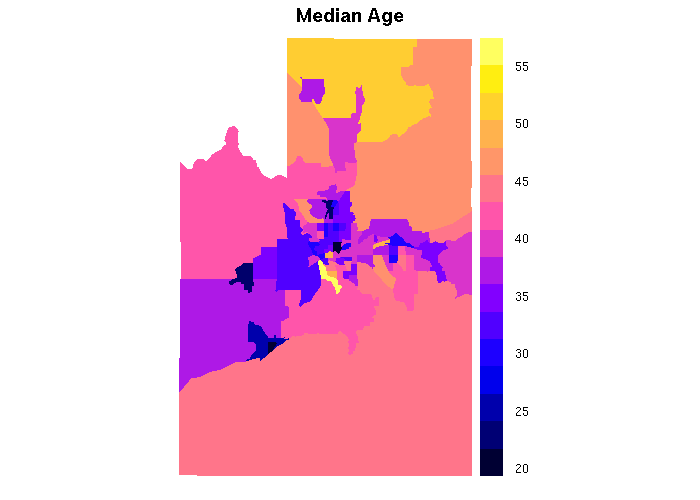Median Age By Census Tract in Spokane County

Neighborhoods in Spokane are used as examples of large polygons Neighborhoods are political boundaries for neighborhood boundaries, which send representatives to the Community Assembly. The assembly agenda for 6 April 2017 included the estimated amount of funds the Community, Housing and Human Services Department (CHHS) allocated to the Neighborhood Community Development Program, Program Year 2018. These grant amounts are presumably need-based and would be proxies for level of community development.

```neighborhoods = readOGR(dsn='.', layer='Neighborhood', stringsAsFactors=F)
ncdp = read.csv('ncdp-grants.csv', stringsAsFactors=F)
neighborhoods = merge(neighborhoods, ncdp, by.x='Name', by.y='Neighborhood', all.x = T)

spplot(neighborhoods, zcol='NCDP', main="NCDP Grants")
```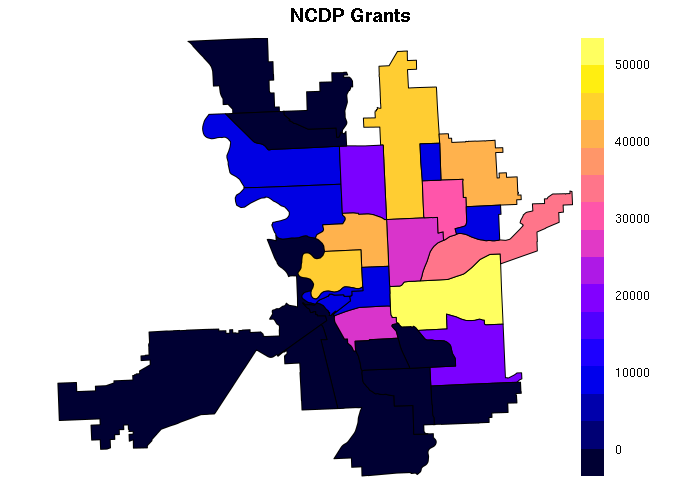NCDP Grants by Neighborhood in Spokane County

Spokane city parks from the Spokane OpenData portal are used as example small polygons:

```parks = readOGR(dsn = '.', layer='Park', stringsAsFactors=F)

plot(neighborhoods, col=NA, border='blue', lwd=3)
plot(parks, col='red', add=T)
```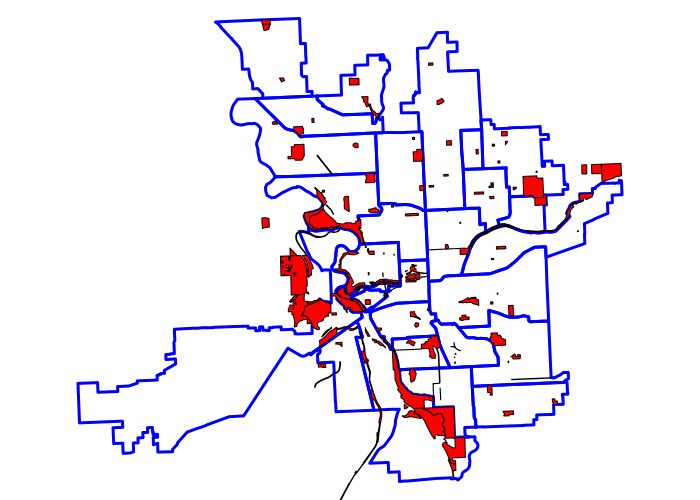City Parks in Spokane, WA

## State Plane Coordinate System

Because spatial operations on local data sets often involve distances in physical length units (like feet) rather than degrees (which vary in ground distance depending on where you are on the planet), it is helpful to use a projection like the US State Plane Coordinate System, which treats an area as a flat surface (plane) and then uses coordinates across that surface expressed in feet or meters. The State Plane Coordinate System is commonly used for geospatial data by local governments that need to be able to measure accurate distances on the ground.

Outside the USA, Universal Transverse Mercator (UTM), which is used by the military and has coordinates in meters, may be your best option.

You can find the appropriate four-digit State Plane Coordinate System FIPS code for your area of analysis using this map. For example, the FIPS code for the northern half of Washington state is 4601.

You can then get the proj4 string by searching with the FIPS code on SpatialReference.org. Choose the appropriate HARN (High Accuracy Reference Network) projection using the NAD 1983 datum (North American Datum).

```state_plane = CRS('+proj=lcc +lat_1=47.5 +lat_2=48.73333333333333
+lat_0=47 +lon_0=-120.8333333333333 +x_0=500000 +y_0=0 +ellps=GRS80 +units=m +no_defs')

parks = spTransform(parks, state_plane)
tracts = spTransform(tracts, state_plane)
neighborhoods = spTransform(neighborhoods, state_plane)

plot(tracts)
plot(neighborhoods, col=NA, border='blue', add=T)
plot(parks, col='red', add=T)
```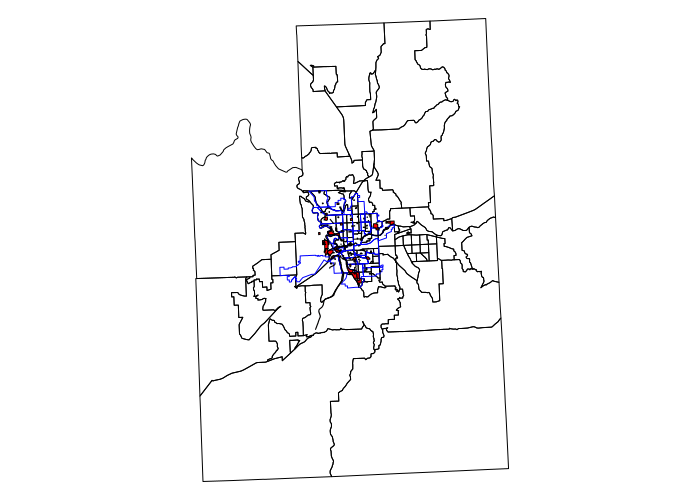City Parks and Census Tracts in Spokane County

## Relationships Between Characteristics in the Same Set Of Areas

If you are analyzing relationships between attributes within the same set of polygons, you can use conventional non-spatial statistical tools like X-Y scatter plot(), cor.test() (correlation test), and lm() (linear modeling) to examine the absence or presence of correlation. While these tools do not deal with issues like spatial autocorrelation that can distort modeling coefficients, these simple functions are especially valuable for exploratory data analysis.

For example, there is an exceptionally strong (and intuitively obvious) correlation between median household income and median monthly housing costs in census tracts:

```> plot(tracts\$MEDHHINC, tracts\$MEDHOUSING)
> print(cor.test(tracts\$MEDHHINC, tracts\$MEDHOUSING))

Pearson's product-moment correlation

data:  tracts\$MEDHHINC and tracts\$MEDHOUSING
t = 19.84, df = 103, p-value < 2.2e-16
alternative hypothesis: true correlation is not equal to 0
95 percent confidence interval:
0.8423540 0.9242395
sample estimates:
cor
0.8902831

> model = lm(MEDHOUSING ~ MEDHHINC + MEDAGE, data=tracts)
> print(summary(model))

Call:
lm(formula = MEDHOUSING ~ MEDHHINC + MEDAGE, data = tracts)

Residuals:
Min      1Q  Median      3Q     Max
-233.56  -50.13  -10.16   60.12  212.76

Coefficients:
Estimate Std. Error t value Pr(>|t|)
(Intercept)  6.141e+02  4.839e+01  12.689  < 2e-16 ***
MEDHHINC     1.082e-02  5.222e-04  20.713  < 2e-16 ***
MEDAGE      -5.905e+00  1.425e+00  -4.144 7.06e-05 ***
---
Signif. codes:  0 ‘***’ 0.001 ‘**’ 0.01 ‘*’ 0.05 ‘.’ 0.1 ‘ ’ 1

Residual standard error: 84.81 on 102 degrees of freedom
Multiple R-squared:  0.8225,    Adjusted R-squared:  0.819
F-statistic: 236.3 on 2 and 102 DF,  p-value: < 2.2e-16
```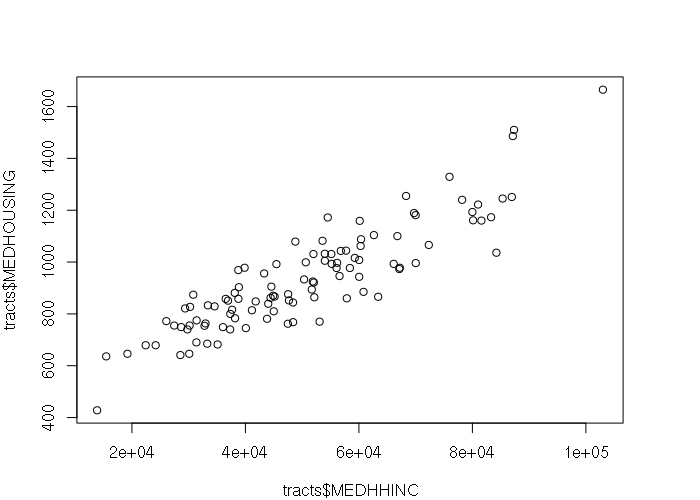Median Household Income vs Median Monthly Housing Cost By Tract In Spokane County, WA

## Aggregation of Smaller Areas Into Larger Ones

When comparing overlapping areas of dissimilar size, some type of operation is usually needed to conceptually either break the larger polygons into smaller areas or aggregate the values from the smaller polygons into the larger areas so the data values between the two areas are spatially comparable.

When aggregating, you will need to make a choice of what kind of aggregation function to use. For counts like population, sum() is the usual choice. For amounts or balances like temperature or latitude, measures of central tendency like mean() or median() are usually better options.

For example, census tracts are smaller than neighborhoods in Spokane County. To get the population of neighborhoods based on population in census tracts, you need to use the over() function to handle population values from tracts that overlap the neighborhoods

• The x parameter is the target features. over() returns one object for each feature in the x layer
• The y parameter is the join features. over() returns values for all features in the join layer that overlap features in the target layer. In this case, we pass features with only the POPULATION attribute since that is all we are concerned with in this operation
• The fn parameter specifies a function that will be applied to all join features that match a single target feature. In this case sum() is used to aggregate a total population for each neighborhood
```neighborhoods\$POPULATION = over(neighborhoods, tracts[,'POPULATION'], fn = sum)\$POPULATION

spplot(neighborhoods, zcol='POPULATION')
```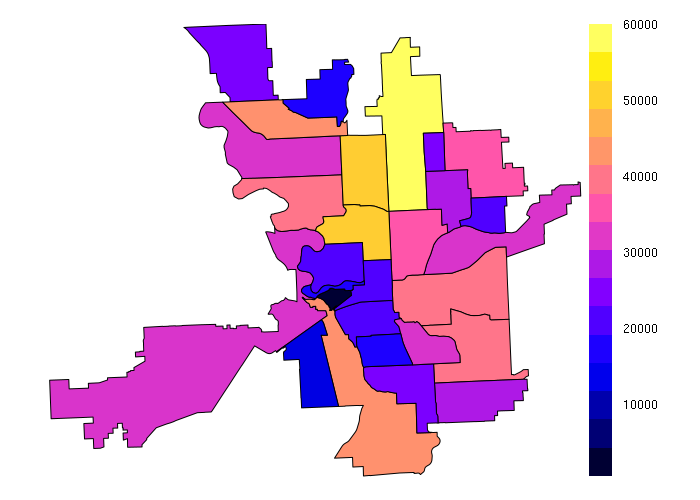Population by Neighborhood Aggregated From Census Tracts

When dealing with counts like population, sometimes density is more useful than absolute values. The area of neighborhoods can be calculated using the gArea() function from the rgeos library, which can then be used to calculate population density, in this case residents per acre. The choropleth shows the comparatively low suburban population for much of the city except for dense settlement in the Peaceful Valley and Whitman neighborhoods.

```library(rgeos)
square_feet_per_acre = 43560
neighborhoods\$AREA = gArea(neighborhoods, byid=T) / square_feet_per_acre
neighborhoods\$DENSITY = neighborhoods\$POPULATION / neighborhoods\$AREA
spplot(neighborhoods, zcol='DENSITY')
```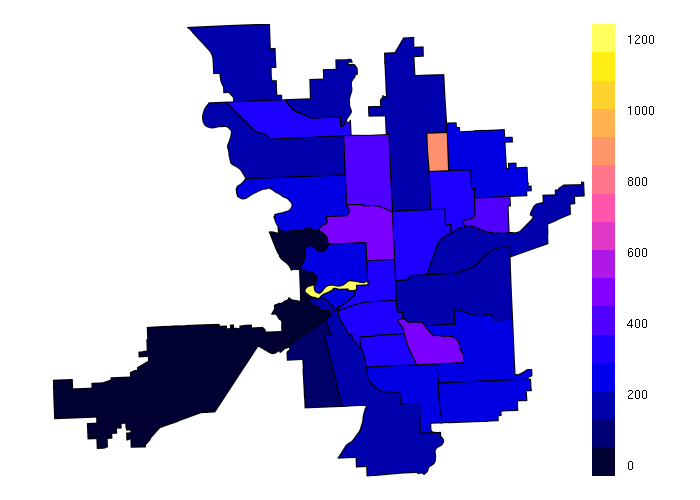Population Density by Neighborhood

With amounts or balances like median household income, mean() is a better choice than sum():

```neighborhoods\$MEDHHINC = over(neighborhoods, tracts[,'MEDHHINC'], fn = mean)\$MEDHHINC

spplot(neighborhoods, zcol='MEDHHINC')
```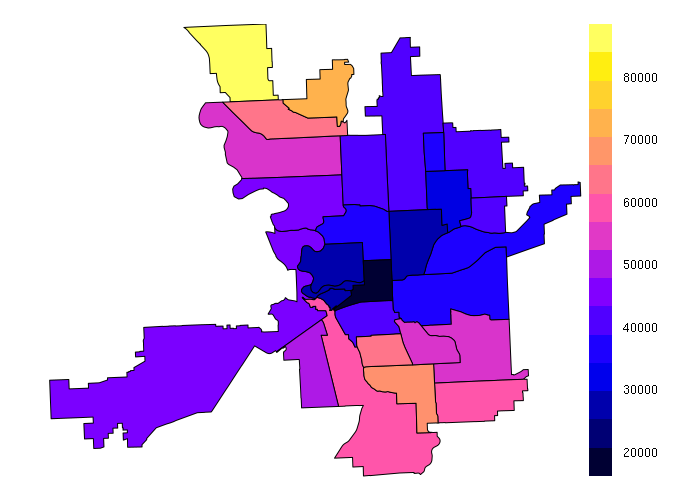Median Household Income by Neighborhood Aggregated From Census Tracts

While aggregation is usually acceptable for counts, aggregation of already aggregated values like median household income adds significant uncertainty to the resulting values.

## Sampling Smaller Areas from Larger Areas

When analyzing dissimilar layers of polygons where the areas of interest are the smaller polygons, over() can be used to sample values from the set of larger polygons.

For example, neighborhoods receive grants from the city's Neighborhood Community Development Program (NCDP). Optimally poorer tracts would be in neighborhoods that receive more NCDP money. We sample values for each of the target layer features from the larger neighborhoods. When multiple join polygons overlap a single target polygon, by default over() selects the first of the overlapping join polygons.

The X-Y scatter plot below shows that indeed, neighborhoods with wealthier tracts receive no NCDP money, but the relationship exhibits heteroskedaciy with poorer tracts in both high-grant neighborhoods and low-grant neighborhoods.

Note that this observation assumes spatial heterogeneity, that the grants are distributed evenly across the neighborhood, but the aggregation of grant money by neighborhood makes it impossible to know whether this unlikely possibility is correct.

```tracts\$NCDP = over(tracts, neighborhoods[,'NCDP'])\$NCDP
plot(tracts\$MEDHHINC, tracts\$NCDP)
```NCDP Grants By Census Tract Sampled From Neighborhoods vs Median Household Income

## Buffers and Areas of Influence

Buffers are polygons created around points, lines or polygons. Buffers represent areas of influence, such as sources or sinks of potential patrons (areas around stores or transit stops).

The concept of buffering is based on the idea encoded in Waldo Tobler's (1979) first law, Everything is related to everything else, but near things are more related than distant things. Buffers are used to identify near things.

Creating a buffer around a feature, overlaying that buffer on top of a target layer of features, and analyzing the presence, number of, or characteristics of target features within the buffers, is one of the most fundamental and powerful analytical tools in GIS.

For these examples, we will consider whether parks are valuable recreational amenities that increase the desirability of residential areas located within walking distance of those parks.

We start with a hypothesis that census tracts located near parks would have higher housing costs than areas further from parks.

To begin to test this hypothesis, we need to use the gBuffer() function from the rgeos library to create 1/4 mile (1,320 foot) buffer polygons around each park to represent the walking distance area of influence:

```library(rgeos)
parkbuffer = gBuffer(parks, byid=T, width=1320)

plot(tracts, xlim=c(753000, 763000), ylim=c(68000, 92000))
plot(parkbuffer, col=NA, border='red', add=T)
plot(parks, col='red', add=T)
```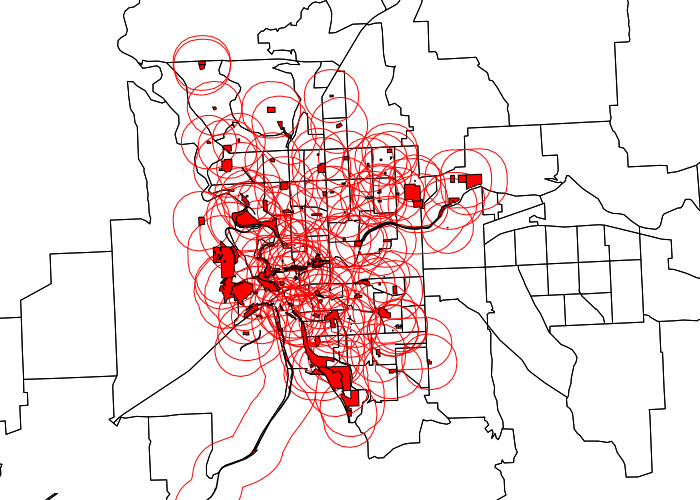Spokane Parks With 1/4-Mile Buffers

## Areas of Influence: Buffering Sources For Presence or Absence

When using buffers to analyze areas influence, the question will arise whether to buffer the source of the influence or the sink of the influence (the objects being influenced).

The buffers create above represent sources of influence, in this case parks as a source of influence on surrounding neighborhoods.

As seen in the map above, the parks data includes a large number of small parks, so that most of the City of Spokane is within walking distance of some kind of park or community facility. However, many of those parks are small spaces with limited recreational value.

To specifically focus on the parks that have such recreational value, we can select parks that are only 10 acres or more (an arbitrary number) using the gArea() function to calculate park area, which is then used to select only large parks for analysis:

```library(rgeos)
square_feet_per_acre = 43560
parks\$ACRES = gArea(parks, byid=T) / square_feet_per_acre

bigparks = parks[parks\$ACRES >= 10,]
bigbuffer = gBuffer(bigparks, byid=T, width=1320)

plot(tracts, xlim=c(753000, 763000), ylim=c(68000, 92000))
plot(bigbuffer, col=NA, border='red', add=T)
plot(bigparks, col='red', add=T)
```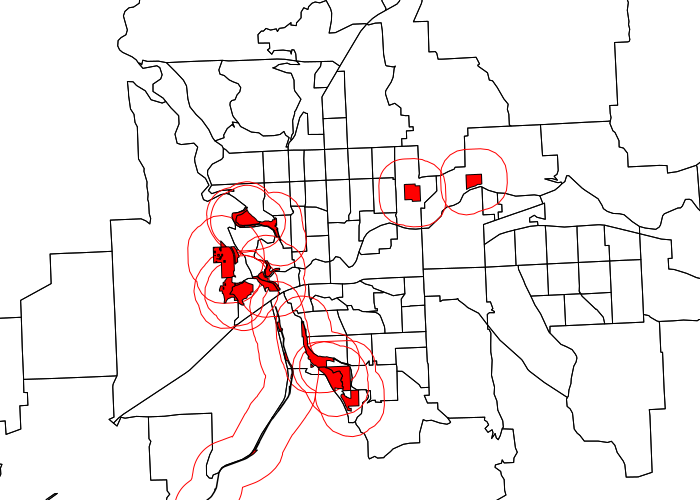Large Spokane Parks With 1/4-Mile Buffers

Buffers like this can be used to flag the presence or absence of influence.

To find the tracts where a significant portion of the tract is within walking distance of at least one large park, we use the gCentroid() function to get the centroids of the census tracts, then use the over() overlay function to set the PARK attribute for each tract to the name of the first park within walking distance. Tracts with no nearby park have a PARK value of NA.

```centroids = gCentroid(tracts, byid=T)
tracts\$PARK = over(centroids, bigbuffer)\$ParkName

col = ifelse(is.na(tracts\$PARK), "white", "lightgray")

plot(tracts, xlim=c(753000, 763000), ylim=c(68000, 92000), col=col)
plot(bigbuffer, col=NA, border='red', add=T)
plot(bigparks, col='red', add=T)
```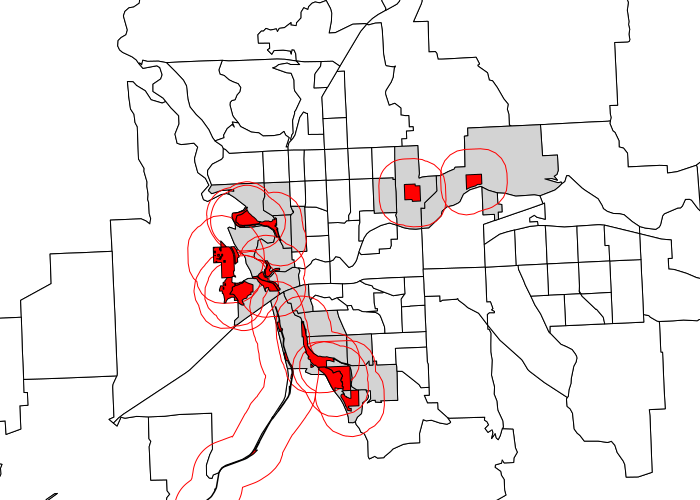Census Tracts In Walking Distance of Large Parks

Listing the median monthly housing costs for all tracts vs just tracts near parks, the housing costs near parks are slightly higher:

```> print("Mean Median Monthly Housing Cost For All Tracts")
> print(mean(tracts\$MEDHOUSING))
 936.6095

> paste("Mean Median Monthly Housing Cost For Tracts Near Parks")
> print(mean(tracts@data[is.na(tracts\$PARK),'MEDHOUSING']))
 942.2955
```

Creating histograms with the hist() function that use the same range (using the breaks parameter), and stacking those histograms on top of each other (by setting the mfrow parameter using the par() function, we can visually compare the distribution of tract values.

```par(mfrow=c(2,1))
hist(tracts\$MEDHOUSING, breaks=seq(400, 1800, 200),
main="All Tracts", xlab = "Median HH Income")
hist(tracts\$MEDHOUSING[!is.na(tracts\$PARK)], breaks=seq(400, 1800, 200),
main="Tracts Near Parks", xlab = "Median HH Income")
```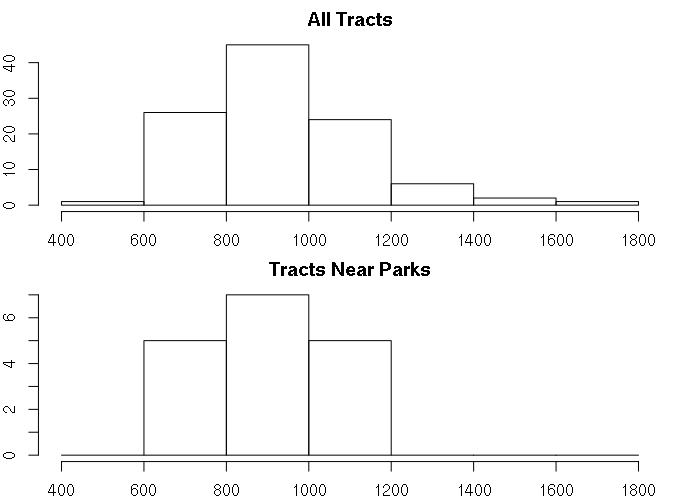Median Median Monthly Housing Costs in All Tracts vs Tracts Near Parks In Spokane County, WA

The histograms look similar and provide no clear evidence that proximity to parks has any significant association with increased housing costs. This indicates that any desirability associated with parks is not significant enough to be reflected in higher housing costs resulting from increased demand.

Accordingly, this is evidence that we should reject our hypothesis that census tracts located near parks would have higher housing costs than areas further from parks.

## Areas of Influence: Buffering Sources For Accessible Totals

While the examples below only assess a binary whether or not the target areas are within areas of influence, source values can also be aggregated for richer analysis.

Continuing with the above example, presumably the larger a park is, the more recreational value it has in terms of walkable/bikeable area. The length of the walk to the park is an inhibitor, but once you get to the park, the full area of the park then becomes valuable.

Using the fn parameter to sum the acreage of accessible parkland:

```library(rgeos)
parkbuffer = gBuffer(parks, byid=T, width=1320)

square_feet_per_acre = 43560
parkbuffer\$ACRES = gArea(parks, byid=T) / square_feet_per_acre

centroids = gCentroid(tracts, byid=T)
tracts\$PARKACRES = over(centroids, parkbuffer[,'ACRES'], fn = sum)\$ACRES
tracts\$PARKACRES[is.na(tracts\$PARKACRES)] = 0

ramp = colorRamp(c("white", "#404040"))
col = rgb(ramp(tracts\$PARKACRES / max(tracts\$PARKACRES)), maxColorValue=255)

plot(tracts, xlim=c(753000, 763000), ylim=c(68000, 92000), col=col)
plot(parks, col='red', add=T)

legend = legend(x = 'bottomright', inset=0.05, pch=15, bg='white',
legend = round(qunif(c(0, 0.5, 1), min=0, max=max(tracts\$PARKACRES))),
col = rgb(ramp(c(0, 0.5, 1)), maxColorValue=255))
```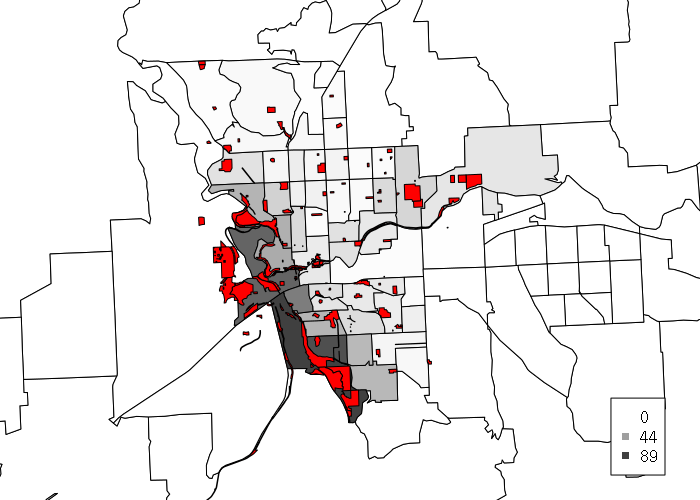Acres of Walk-Accessible Parkland By Tract

Plotting an X/Y-scatter of walk-accessible parkland vs median monthly housing costs shows no correlation, further corroborating the conclusion that parks are not an amenity that increase the significantly increase housing costs in adjacent neighborhoods.

```plot(tracts\$MEDHOUSING, tracts\$PARKACRES)
```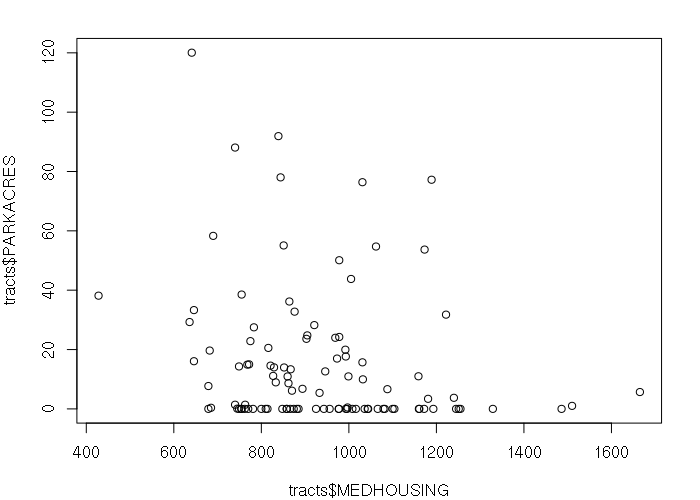Acres of Walkable Parkland vs Median Household Income

## Areas of Influence: Sink to Source

The choice of whether to buffer the source or the sink associated with an area of influence is dependent upon the particular situation, and in some cases may yield similar results. One approach may also require less coding than the other.

This example buffers the sink of influence (people) rather than the source of influence (parks).

From the standpoint of a walker, the size of the accessible parks may be less relevant than the amount of parkland that is easily accessible. If a person only walks a couple of miles a day, having access to a 10-mile trail is no more valuable tha access to a 10-acre park with a pleasant circular walking path.

To perform this analysis requires creating an intersection of a buffer around each tract and all parks within that buffer to calculate only the park area in walking distance. An intersection is all polygons or portions of polygons in the target layer that entirely contained by a feature in the join layer.

```tractbuffers = gBuffer(centroids, byid=T, width=2640)

intersected_parks = gIntersection(parks, tractbuffers[2,])

plot(parks, xlim=c(753000, 763000), ylim=c(68000, 92000), col='lightgray')
plot(tractbuffers[2,], border='blue', add=T)
plot(intersected_parks, col='red', add=T)
```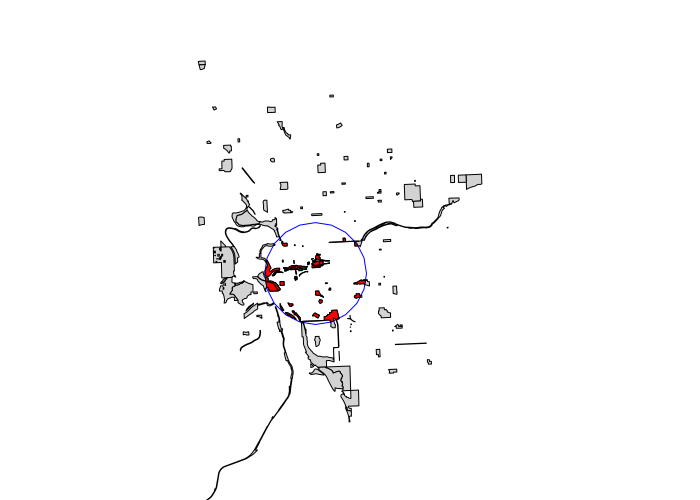An Example of Intersection

The script below:

• Creates buffers around the centroids of census tracts
• Uses sapply() to performs operations on each tract buffer
• Uses gIntersection() with each tract buffer and the parks layer to find the park area that intersects the tract buffer
• Uses gArea() to find the total area of those intersecting parks, representing the total park area within 1/2 mile of the center of each census tract, or a convenient after-dinner walk
• Returns that total park area for each tract so sapply() can set the PARK area column in the tracts data
```tractbuffers = gBuffer(centroids, byid=T, width=2640)

tracts\$PARKACRES = sapply(1:length(tractbuffers), function(z) {
x = gIntersection(parks, tractbuffers[z,])
return(ifelse(is.null(x), 0, gArea(x) / 43560)) })

ramp = colorRamp(c("white", "#404040"))
col = rgb(ramp(tracts\$PARKACRES / max(tracts\$PARKACRES)), maxColorValue = 255)

plot(tracts, xlim=c(753000, 763000), ylim=c(68000, 92000), col=col)
plot(parks, col='red', add=T)
legend(x="bottomright", col=rgb(ramp(c(0, 0.5, 1)), maxColorValue=255),
legend = c(0, round(max(tracts\$PARKACRES) / 2), round(max(tracts\$PARKACRES))),
inset=0.05, bg="white", pch=15)
```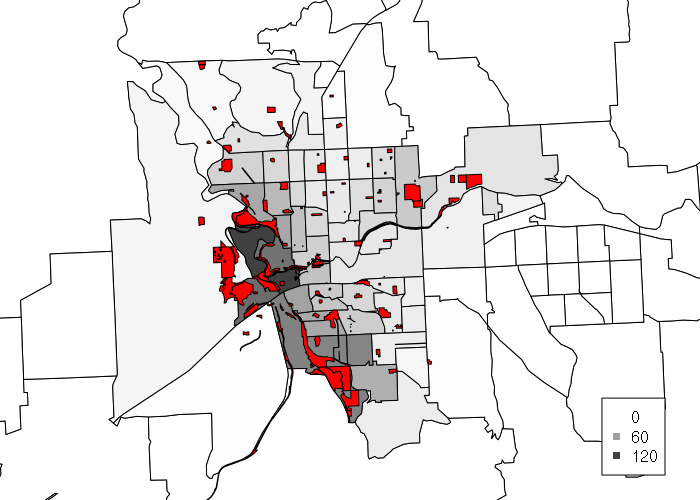Acres of Walkable Parkland By Tract

As might be expected, the choropleth from the buffered sinks accentuates areas where parkland is more densely located.

Plotting an X/Y-scatter of walkable parkland vs median monthly housing costs shows no correlation, further corroborating the conclusion that parks are not an amenity that significantly increase housing costs in adjacent neighborhoods.

```plot(tracts\$MEDHOUSING, tracts\$PARKACRES)
```Acres of Walkable Parkland vs Median Household Income

## Limitations

Note that while buffering is powerful, it also has some limitations.

Buffers often assume that space is homogeneous and that features are evenly distributed within areas. However, when features are aggregated within zones (such as median household income in census tracts), you should be careful to avoid the environmental fallacy in assuming that all of those features have the value of central tendency.

Features that are aggregated and summarized within zones may also be clustered unevenly within those zones, such as with isolated neighborhoods surrounding large areas of uninhabited land in rural census tracts, or a handful of dispersed residents in urban industrial areas. For example, if a buffer overlaps half of the area of a census tract, you cannot be assured that the buffer covers half of the population.

Distance is especially tricky with buffers since travelers must navigate along roads and between structures travel may not be in a straight line. So 1/2 mile in Euclidian distance (as the crow flies) may be longer if there is not a direct path on the ground from source to destination. This is especially notable when there are physical barriers like highways, water bodies, fences or hills. Advanced GIS software can calculate distance and travel time using road and sidewalk networks, although such calculations are more complex than simple buffering and involve access to complex and often-changing road network data hosted on servers and costing money to access.